## Two Examples of Localized Wave Packets

Lets now try two examples of a wave packet localized in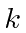and properly normalized at.
1. A square'' packet:for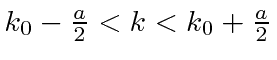and 0 elsewhere.
2. A Gaussian packet:.

These are both localized in momentum about.

Check the normalization of (1).Check the normalization of (2) using the result for a definite integral of a Gaussian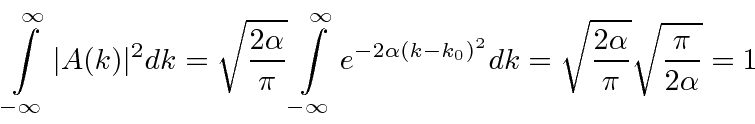So now we take the Fourier Transform of (1) right here.Note thatis equal to 1 atand that it decreases from there. If you square this, it should remind you of a single slit diffraction pattern! In fact, the single slit gives us a square localization in position space and the F.T. is this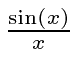function.

The Fourier Transform of a Gaussian wave packet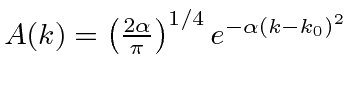isalso a Gaussian. We will show later that a Gaussian is the best one can do to localize a particle in position and momentum at the same time.

In both of these cases of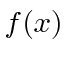(transformed from a normalizedlocalized in momentum space) we see

• A coefficient which correctly normalizes the state to 1,
•- a wave corresponding to momentum,
• and a packet function which is localized in.

We have achieved our goal of finding states that represent one free particle. We see that we can have states which are localized both in position space and momentum space. We achieved this by making wave packets which are superpositions of states with definite momentum. The wave packets, while localized, have some width inand in.

Jim Branson 2013-04-22